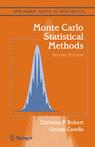Want to share your content on R-bloggers? click here if you have a blog, or here if you don't.An X’idated reader of Monte Carlo Statistical Methods had trouble with our Example 3.13, the very one our academic book reviewer disliked so much as to “diverse [sic] a 2 star”. The issue is with computing the integral$\mathfrak{I}=\int\limits_{-\infty}^{\infty}{\sqrt{\left|\frac{\theta}{1-\theta}\right|}}f(\theta)\text{d}\theta$

when f is the Student’s t(5) distribution density. In our book, we compare a few importance sampling solutions, but it seems someone (the instructor?) suggested to this X’idated reader to use a mixture importance density$0.5\{{{g}_{1}}(\theta )+{{g}_{2}}(\theta )\},$

with${{g}_{1}}(\theta )=\dfrac{1}{\pi }\dfrac{1}{1+{{\theta}^{2}}}$

and${{g}_{2}}(\theta )=\dfrac{1}{4\sqrt{\left|1-\theta\right|}}\quad\text{on}\quad[0,2]$

i.e. with a Cauchy and a power component. The suggested solution in our book is a folded Gamma distribution$g^\prime(\theta)\propto\dfrac{1}{\sqrt{\left|1-\theta\right|}}\exp (-\left|1-\theta\right|).$

The above graph is my final answer to this X’idated reader (after several exchanges in the comments), comparing the three solutions in terms of variability. I used

> m=function(x){
+ sqrt(abs(x/{1-x}))*dt(x,df=5)}
> sam1=matrix(rt(10^7,df=5),ncol=100)
> fam1=m(sam1)


for the first box (the matrix is used for computing 100 replicas),

> g=function(x){
+ .5*dcauchy(x)+.125*((x>0)*(x<2))/sqrt(abs(1-x))}
> sam22=1+sample(c(-1,1),5*10^6,rep=TRUE)*runif(5*10^6)^2
> sam21=rcauchy(5*10^6)
> sam2=matrix(sample(c(sam21,sam22)),ncol=100)
> fam2=m(sam2)*dt(sam2,df=5)/g(sam2)


for the second box (with the details that$F(x)=\begin{cases}\dfrac{1}{2}(1-\sqrt{1-x}) &\text{if } 0\le x\le 1\\ \dfrac{1}{2}(1+\sqrt{x-1}) &\text{if } 1\le x\le 2\\\end{cases}$

is the cdf associated with the second component and that it can be easily inverted into 1±u² for simulation,

> sam3=matrix(1+sample(c(-1,1),5*10^7,
+ rep=TRUE)*rgamma(10^7,.5),ncol=100)
> fam3=m(sam3)*dt(sam3,df=5)/(.5*dgamma(abs(1-sam3),.5))for the third box. The gradual improvement brought by importance sampling is clear there. Which makes me think we should present the comparison that way in the next edition of Monte Carlo Statistical Methods.

Filed under: Books, Kids, R, Statistics, University life Tagged: cross validated, importance sampling, Monte Carlo methods, Monte Carlo Statistical Methods, simulation